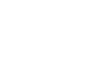what is difference between DC and AC? - letsdiskussRIYA KUMARI

student | Posted | Education

# what is difference between DC and AC?

19
0

Major difference between AC and DC voltage

The major distinction between AC and DC voltage is that the polarity of the wave varies with time in AC voltage, whereas the polarity of DC voltage never changes. The comparison chart below shows the various differences between AC and DC voltage.

Basis for Comparison AC voltage direct current voltage

Definition AC voltage is the force that derives the alternating current between the two points. DC voltage induces direct current between the two points.

Symbolic RepresentationFrequency Depends on the country. Zero
Power factor Lies between 0 and 1. 0
Polarity Change remains constant
Direction Vary stay even
obtained from Generator Cell or Battery
Efficiency High Low
Passive Parameter Impedance Resistance
Amplitude To have Do not have
Conversion Using the inverter. Using the rectifier.
Transformer Requires for transmission. Does not require.
Phase and Neutral To have Do not have
Benefits Easy to measure. amplify easily

AC voltage definition

The AC voltage is the voltage that creates alternating current. When the current conductor rotates in the magnetic field, it induces alternating current in the coil. When the conductor rotates, the magnetic flux is cut off, and the flux variation causes the conductor to produce alternating voltage.

DC voltage definition
Direct current is induced by DC voltages. Waves in only one direction, with the magnitude of the voltage remaining constant. DC voltage generation is a simple and straightforward process. The voltage generates rotation of the coil in the magnet's field. The split ring and the commutator make up the coil, which transforms alternating voltage to forward voltage.

Main differences between AC and DC voltage
The voltage that causes alternating current is known as alternating voltage. DC voltage produces direct current.
The frequency of AC voltage depends on the country (usually 50 and 60 Hz are used). Whereas, the DC voltage frequency becomes zero.
The power factor for AC voltage is between 0 and 1. And the power factor for DC voltages always remains 1.
The polarity of the AC voltage always varies with time, and the polarity of the DC voltage always remains constant.
AC voltage is unidirectional and DC voltage is bidirectional.
The generator generates AC voltage and DC voltage is taken from the cell or battery.
The efficiency of AC voltage is high compared to DC voltage.
Impedance is the passive parameter of AC voltage, and for DC it is resistance. Impedance means the opposition offered by voltage to current flow.
AC voltage has amplitude, while DC voltage has no amplitude. The term amplitude means the maximum distance covered by the oscillation and vibrating body.
The inverter converts direct current into alternating current. Whereas, the rectifier converts alternating current into direct current.
The transformer is essential for AC transmission while not being used for DC transmission.
AC voltage has phase and neutral, while DC voltage does not require phase or neutral.
The main advantage of alternating voltage is that it is easily measured. The advantage of DC voltage is that the voltage can be easily amplified. Amplification is the process by which signal strength increases.

Relationship between AC and DC voltage
AC Volts Χ 1.414 = DC Volts

29
0

Content writer | Posted

AC and DC describe types of current flow in an electric circuit . In Alternating Current (AC) the direction of the flow of electricity changes direction to and fro at regular intervals while in Direct current (DC) electrical current flows consistently in one direction.For Ac few substations are required for generating and transmitting but for DC more substations are required. In AC raising and lowering of voltage is easier so it is used in factories,industries and residential areas .DC power is used for applications where the need is of low voltage and some of them are like charging batteries, automotive applications. .All solar panels nowadays produce DC power.0
0